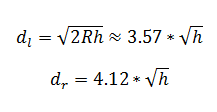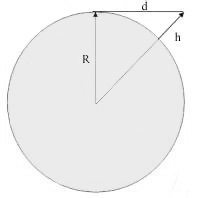# Line of Sight Calculator

This CalcTown line of sight calculator calculates how far the horizon is from an antenna placed at a particular height. The calculators also calculates the radio horizon. It assumes there is nothing between the radio signals and the antenna horizon. To get maximum line of distance you need to enter the height of the antenna.

#### Result

km
kmWhere,

h = height of the antenna

R = radius of the earth

dl = total line of sight

dr = radio horizon (actual service range)